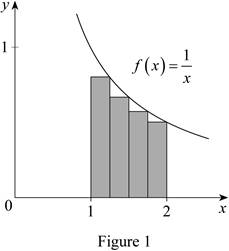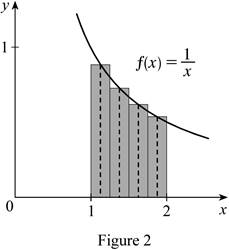# The Riemann sum for the function f ( x ) = 1 x . To represent: The Riemann sum for the function f ( x ) = 1 x .### Single Variable Calculus: Concepts...

4th Edition
James Stewart
Publisher: Cengage Learning
ISBN: 9781337687805### Single Variable Calculus: Concepts...

4th Edition
James Stewart
Publisher: Cengage Learning
ISBN: 9781337687805

#### Solutions

Chapter 5.2, Problem 4E

(a)

To determine

## To find: The Riemann sum for the function f(x)=1x.To represent: The Riemann sum for the function f(x)=1x.

Expert Solution

The Riemann sum for the function f(x)=1x is 0.634524_.

The Riemann sum for the function represents the sum of the area of rectangles with respect to the x-axis.

### Explanation of Solution

Given information:

The function is f(x)=1x

The region lies between x=1 and x=2.

Number of rectangles is n=4.

Find the width (Δx) using the relation:

Δx=ban (1)

Substitute 2 for b, 1 for a, and 4 for n in Equation (1).

Δx=214=14

Determine right end points xi using the relation:

xi=a+(i)Δx (2)

Calculate the value of x¯i for four subintervals:

Substitute 1 for a, 1 for i,and 14 for Δx in Equation (2).

x¯1=1+(1)×(14)=1+14=54

Substitute 1 for a, 2 for i,and 14 for Δx in Equation (2).

x¯2=1+(2)×(14)=1+12=32

Substitute 1 for a, 3 for i, and 14 for Δx in Equation (2).

x¯3=1+(3)×(14)=1+34=74

Substitute 0 for a, 4 for i,and 14 for Δx in Equation (2).

x¯4=1+(4)×(14)=1+1=2

Substitute x¯i for x in the function f(x)=1x.

f(x¯i)=1x¯i (3)

Calculate the value of f(x¯i) using Equation (3).

Substitute 54 for x¯1 in Equation (3).

f(x¯1)=1(54)=45

Substitute 32 for x¯2 in Equation (3).

f(x¯2)=1(32)=23

Substitute 74 for x¯3 in Equation (3).

f(x¯3)=1(74)=47

Substitute 2 for x¯4 in Equation (3).

f(x¯4)=12

The expression to find Riemann sum of areas of n rectangles (Ln) is shown below:

Rn=i=1nf(x¯)Δx=f(x¯1)Δx+f(x¯2)Δx+...+f(x¯n)Δx (4)

Here, the midpoint height of the first rectangle is f(x¯1), the width is Δx, the midpoint height of the second rectangle is f(x¯2), and the midpoint height of the nth rectangle is f(x¯n).

Draw six rectangles using mid points for the function f(x)=1x as shown in Figure 1.Substitute 14 for Δx,(45) for f(x¯1), (23) for f(x¯2), (47) for f(x¯3),and (12) for f(x¯4), in Equation (4).

Mn=Δx[f(x¯1)+f(x¯2)+f(x¯3)+f(x¯4)]=14[45+23+47+12]=14(2.5381)0.634524

Therefore, the Riemann sum for the function f(x)=1x using mid points is 0.634524_.

Show the Representation of Riemann sum:

Refer to Figure 1.

The Riemann sum represents the sum of area of four rectangles above the x-axis.

Thus, the Riemann sum for the function represents the net area of the rectangles with respect to its axis.

(b)

To determine

### To find: The Riemann sum for the function f(x)=1x.To represent: The Riemann sum for the function f(x)=1x.

Expert Solution

The Riemann sum for the function f(x)=1x is 0.691220_.

The Riemann sum for the function represents the sum of the area of rectangles with respect to the x-axis.

### Explanation of Solution

Given information:

The function is f(x)=1x.

The region lies between x=1 and x=2.

Number of rectangles is n=4.

Find the width (Δx) using the relation:

Δx=ban (5)

Substitute 2 for b, 1 for a, and 4 for n in Equation (5).

Δx=214=14

Calculate the value of xi using relation:

xi=a+iΔx (6)

Calculate the value of xi1 using Equation (6)

Substitute (i1) for i in Equation (2)

xi1=a+(i1)Δx (7)

Calculate the value of mid points using the relation:

x¯i=12(xi1+xi) (8)

Substitute (a+iΔx) for xi and [a+(i1)Δx] for xi1 in Equation (8)

x¯i=12[a+(i1)Δx+a+iΔx]=12(a+iΔxΔx+a+iΔx)=12×[2(a+iΔx)Δx]=(a+iΔx)Δx2 (9)

Calculate the value of x¯i for four subintervals:

Substitute 1 for a, 1 for i,and 14 for Δx in Equation (9).

x¯1=(1+1×14)12×(14)=5418=98

Substitute 1 for a, 2 for i,and 14 for Δx in Equation (9).

x¯2=(1+2×14)12×(14)=3218=118

Substitute 1 for a, 3 for i,and 14 for Δx in Equation (9).

x¯3=(1+3×14)12×(14)=7418=138

Substitute 1for a, 4 for i,and 14 for Δx in Equation (9).

x¯2=(1+4×14)12×(14)=218=158

Substitute x¯i for x in the function f(x)=1x.

f(x¯i)=1x¯i (10)

Calculate the value of f(x¯i) using Equation (10).

Substitute 98 for x¯1 in Equation (10).

f(x¯1)=1(98)=89

Substitute 118 for x¯2 in Equation (10).

f(x¯2)=1(118)=811

Substitute 138 for x¯3 in Equation (10).

f(x¯3)=1(138)=813

Substitute 2 for x¯4 in Equation (10).

f(x¯4)=1(158)=815

The expression to find Riemann sum (Mn) of areas of n rectangles using mid points is shown below.

Mn=i=1nf(x¯)Δx=f(x¯1)Δx+f(x¯2)Δx+...+f(x¯n)Δx (11)

Here, the midpoint height of the first rectangle is f(x¯1), the width is Δx, the midpoint height of the second rectangle is f(x¯2), and the midpoint height of the nth rectangle is f(x¯n).

Draw four rectangles using mid points for the function f(x)=1x as shown in Figure 1.Substitute 14 for Δx,(89) for f(x¯1), (811) for f(x¯2), (813) for f(x¯3),and (815) for f(x¯4) in Equation (11).

Mn=Δx[f(x¯1)+f(x¯2)+f(x¯3)+f(x¯4)]=14[89+811+813+815]=12(2.7648796)=0.691220

Show the Representation of Riemann sum:

Refer to Figure 1.

The Riemann sum represents the sum of area of four rectangles above the x-axis.

Thus, the Riemann sum for the function represents the net area of the rectangles with respect to its axis.

### Have a homework question?

Subscribe to bartleby learn! Ask subject matter experts 30 homework questions each month. Plus, you’ll have access to millions of step-by-step textbook answers!